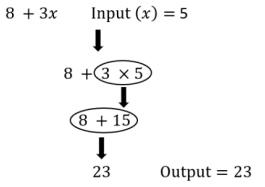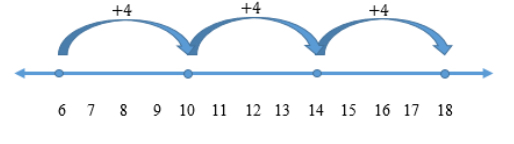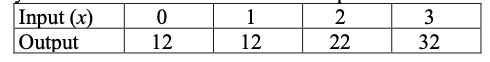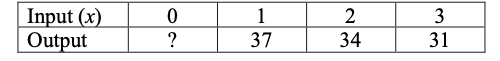# MA.5.AR.3.2Export Print
Given a rule for a numerical pattern, use a two-column table to record the inputs and outputs.

### Examples

The expression 6+2x, where x represents any whole number, can be represented in a two-column table as shown below.

 Input (X) 0 1 2 3 Output 6 8 10 12

### Clarifications

Clarification 1: Instruction builds a foundation for proportional and linear relationships in later grades.

Clarification 2: Rules are limited to one or two operations using whole numbers.

General Information
Subject Area: Mathematics (B.E.S.T.)
Strand: Algebraic Reasoning
Status: State Board Approved

## Benchmark Instructional Guide

• NA

### Vertical Alignment

Previous Benchmarks

Next Benchmarks

### Purpose and Instructional Strategies

The purpose of this benchmark is to relate patterns to a two-column table for students to record inputs and outputs. It is related to MA.5.AR.3.1 where students determine rules from given patterns. This is the first grade in which students record inputs and outputs two-column tables, and this work helps build the foundation for proportional relationships (MA.6.AR.3.3 and MA.7.AR.4) in middle school and functional relationships starting in grade 8.
• Instruction of this benchmark should be paired with MA.5.AR.3.1. Organizing patterns into input and output tables lays the foundation for students to explore proportional and linear relationships in later grades (MTR.5.1).
• During instruction, teachers can relate the idea of “inputs” and “outputs” on a two- column table to a machine. The input is the term number, and the output is the corresponding term’s value. Students are to find what the machine does to determine the output.
• Instruction should make connections between representing the information in a two- column table and as ordered pairs on a coordinate plane (MA.5.GR.4.2).

### Common Misconceptions or Errors

• Students may make computational errors when calculating the output for a given rule and input.
• Students may confuse input and output values when recording the values in a two- column table.

### Strategies to Support Tiered Instruction

• Instruction includes opportunities to record each step when calculating the output for a given rule and input.
• For example, for the rule 8 + 3x students record the steps to calculate the output using an input of 5 and the order of operations.• Instruction includes using highlighters when recording inputs and outputs in a two- column table. Students highlight the “inputs” label in the table and all corresponding inputs using one color. Then, students highlight the “outputs” label in the table and all corresponding outputs using a different color.The Math Machine makes two-column tables when the user tells it a rule. Jacob tells the Math Machine to create a table using the rule “10 + 2x.” Unfortunately, the machine is malfunctioning and only some of the table is correct.
• Part A: Identify which values are incorrect and complete the table correctly.• Part B: Extend your table to show the outputs for x = 10, 11 and 12.

### Instructional Items

Instructional Item 1

What is the missing value in the two-column table below?
Rule: 40 − 3x*The strategies, tasks and items included in the B1G-M are examples and should not be considered comprehensive.

## Related Courses

This benchmark is part of these courses.
5012070: Grade Five Mathematics (Specifically in versions: 2014 - 2015, 2015 - 2022, 2022 and beyond (current))
7712060: Access Mathematics Grade 5 (Specifically in versions: 2014 - 2015, 2015 - 2018, 2018 - 2022, 2022 and beyond (current))
5012065: Grade 4 Accelerated Mathematics (Specifically in versions: 2019 - 2022, 2022 and beyond (current))
5012015: Foundational Skills in Mathematics 3-5 (Specifically in versions: 2019 - 2022, 2022 and beyond (current))

## Related Access Points

Alternate version of this benchmark for students with significant cognitive disabilities.
MA.5.AR.3.AP.2: Given the inputs and a one-step addition or subtraction rule for a numerical pattern, use a two-column table to record the outputs.

## Related Resources

Vetted resources educators can use to teach the concepts and skills in this benchmark.

## Formative Assessments

Choo Choo Trains Company:

Students are asked to fill in missing values in a table of numerical patterns and describe relationships between corresponding terms.

Type: Formative Assessment

Comic Books:

Students are asked to complete one of two number patterns, write ordered pairs composed of corresponding terms, graph the ordered pairs, and identify a relationship between corresponding terms of the patterns.

Type: Formative Assessment

## Lesson Plans

Cool School:

In this lesson, students will take an imaginary trip to my lake house. They will solve a variety of real life word problems. Once at the lake they will experience many activities where they will need number sense. For the assessment, students will record and analyze data in order to determine who the best angler was at "Cool School."

Type: Lesson Plan

Gummy vs. Gum (Number Pattern):

In this lesson from the Beacon Learning Center, students use gummy bears and sticks of gum to discover a number pattern and write an representation that describes it.

This lesson can be adapted for several grade levels and instead of writing an equation, students can write a "rule" or expression based on the pattern or relationship between number of pieces of gum and gummy bears.  Consider scaffolding this activity to meet your grade-level needs.

Type: Lesson Plan

## Tutorial

Number Pattern Example: Growing Sequence:

In this Khan Academy tutorial video a table is used to track a growing sequence of design.

Type: Tutorial

## MFAS Formative Assessments

Choo Choo Trains Company:

Students are asked to fill in missing values in a table of numerical patterns and describe relationships between corresponding terms.

Comic Books:

Students are asked to complete one of two number patterns, write ordered pairs composed of corresponding terms, graph the ordered pairs, and identify a relationship between corresponding terms of the patterns.

## Student Resources

Vetted resources students can use to learn the concepts and skills in this benchmark.

## Tutorial

Number Pattern Example: Growing Sequence:

In this Khan Academy tutorial video a table is used to track a growing sequence of design.

Type: Tutorial

## Parent Resources

Vetted resources caregivers can use to help students learn the concepts and skills in this benchmark.